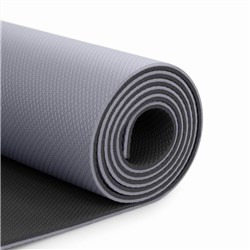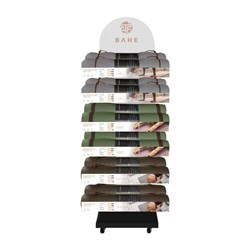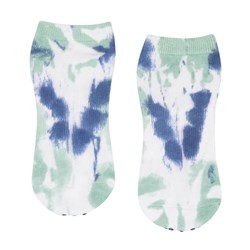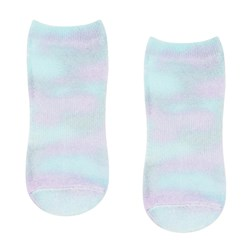# Pilates & Yoga

Grid List
\$29.99
Increase value Decrease value
\$39.99
Increase value Decrease value\$44.00
Increase value Decrease value
\$59.99
Increase value Decrease value
BAHE Yoga Wheel
In Stock
\$69.99
Increase value Decrease value
\$19.99
Increase value Decrease value
\$34.99
Increase value Decrease value\$499.00
Increase value Decrease value
\$16.25
Increase value Decrease value
\$49.96
Increase value Decrease value
\$19.00
Increase value Decrease value
POW.R Yoga Brick
In Stock
\$19.99
Increase value Decrease value
\$6.99
Increase value Decrease value
POW.R Yoga Mat
In Stock
\$19.99
Increase value Decrease value
POW.R Yoga Wheel
In Stock
\$39.99
Increase value Decrease value
In Stock
\$47.85
Increase value Decrease value
From
Increase value Decrease value
From
Increase value Decrease value
From
Increase value Decrease value
From
Increase value Decrease value
From
Increase value Decrease value
From
Increase value Decrease value
From
Increase value Decrease value
From
Increase value Decrease value
From
Increase value Decrease value
From
Increase value Decrease value
From
Increase value Decrease value
From
Increase value Decrease valueFrom
Increase value Decrease valueFrom
Increase value Decrease value
From
Increase value Decrease value
From
Increase value Decrease value
From
Increase value Decrease value
From
Increase value Decrease value
Grid List
##### Products to compare:
Comparing Products